# Gold, Silver, and Bronze Proportioned Papers

In mathematics, art, and origami (which is a combination of both), you will sometimes come upon the concept of a gold rectangle, a silver rectangle, or a bronze rectangle. What do all these mean? These are simply names which define the specific shape of paper.

 Name Bronze Rectangle Silver Rectangle Golden Rectangle Ratio of Sides 1:√3 1:√2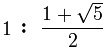Make One here here or here here or here, or here
Comparatively, the US letter sized paper is the most stumpy: it is wide and short. The silver rectangle (1 to 1.41) is similar to US Letter size except it is a little taller/skinnier. The gold rectangle (ratio 1 to 1.61) is even more taller, and the bronze rectangle (ratio 1 to 1.73) is the tallest rectangle in the image below.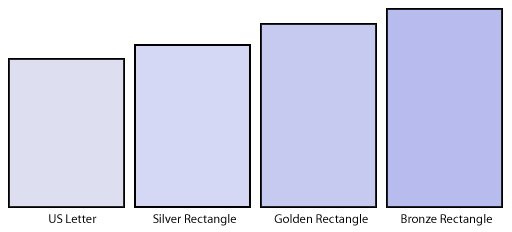#### Silver Rectangles

The International Standard A-series papers are used around the world with the exception of USA and Canada. The A-series papers are examples of silver rectangles and have width to length ratios of 1 to √2. These rectangles are special because if you cut the paper in half crosswise, the two resulting pieces are also of the ratio 1:√2.

For example: A4 paper is 8.27″ × 11.69″ which is of the ratio 1 to √2. If you cut the A4 in half, the pieces are now called A5 and their sizes are 5.83″ × 8.27″ (note how the width is now the length). The ratio of A5 is also 1:√2.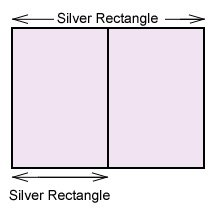You can cut the paper in half repeatedly and the resulting rectangles will all have the silver ratio. Some argue that this unique feature makes the International Standard paper sizes (A, B and C series) more versatile and superior compared to the US Letter sized paper.

#### Bronze Rectangles

The ratio of the width to length of a bronze rectangle is 1:√3. In origami, bronze rectangles are often used for making shapes with 30°, 60° or 120° angles. For example, origami equilateral triangles, tetrahedra, and icosahedra often use the creases from a bronze rectangle. You do not need to start with a piece of bronze rectangle; it is sufficient if you fold the bronze rectangle into the existing paper.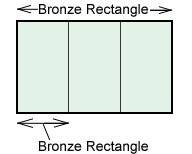If you cut the bronze rectangle into thirds, the resulting pieces are also of the ratio 1:√3. In this manner, the silver and bronze rectangles are useful.

#### Golden Rectangle

The golden rectangle is not used very much in origami; however, it is added here for completion. The ratio of the sides is 1:1.61 or in fraction format: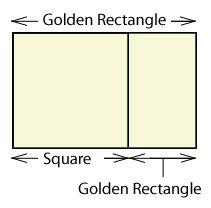The special feature of the golden rectangle is that if you remove a square from the rectangle, the left-over shape is also a golden rectangle with ratio 1:1.61.

The golden rectangle is used in art because it is thought to be visually pleasing and harmonious. The golden rectangle is also found in nature.